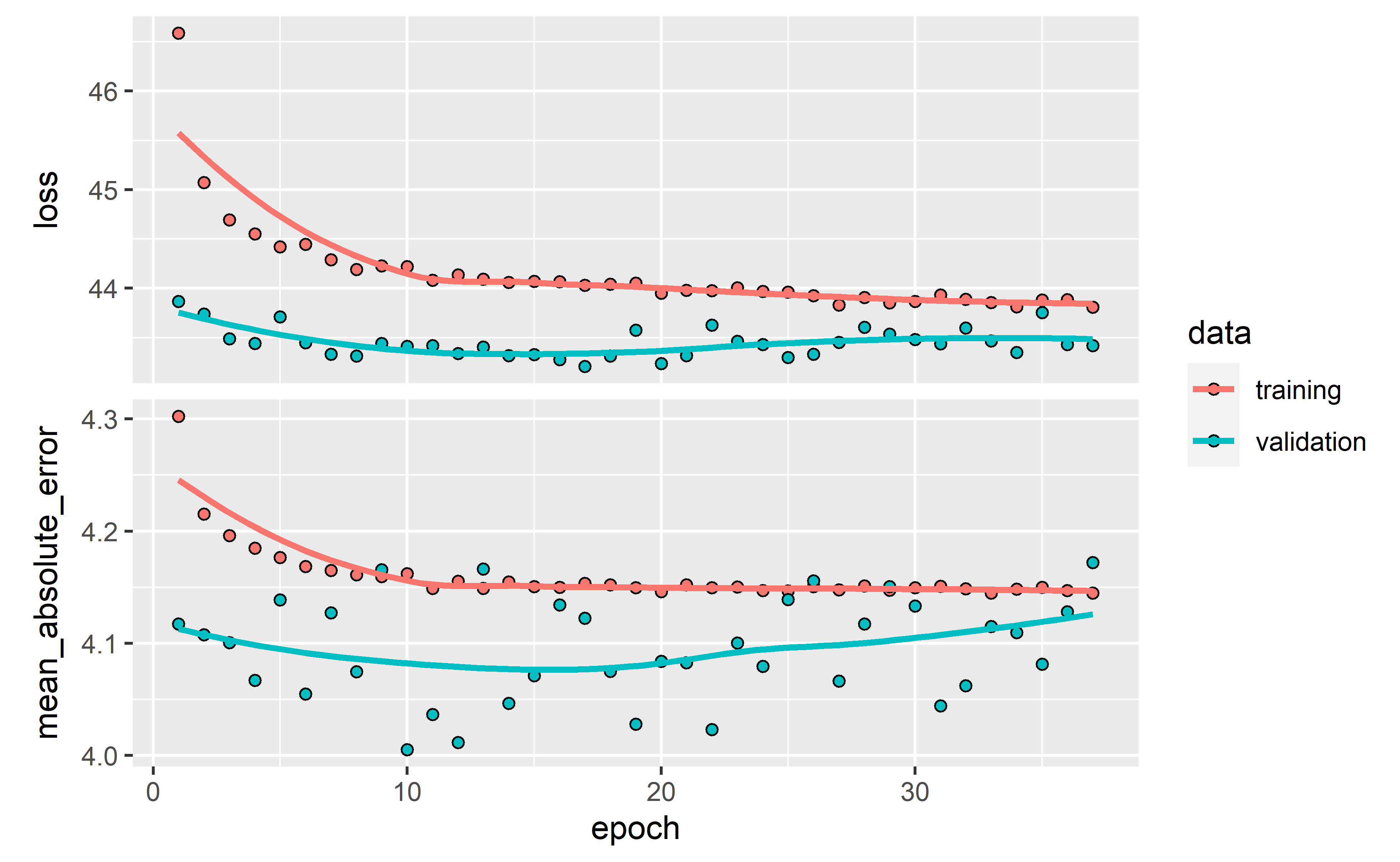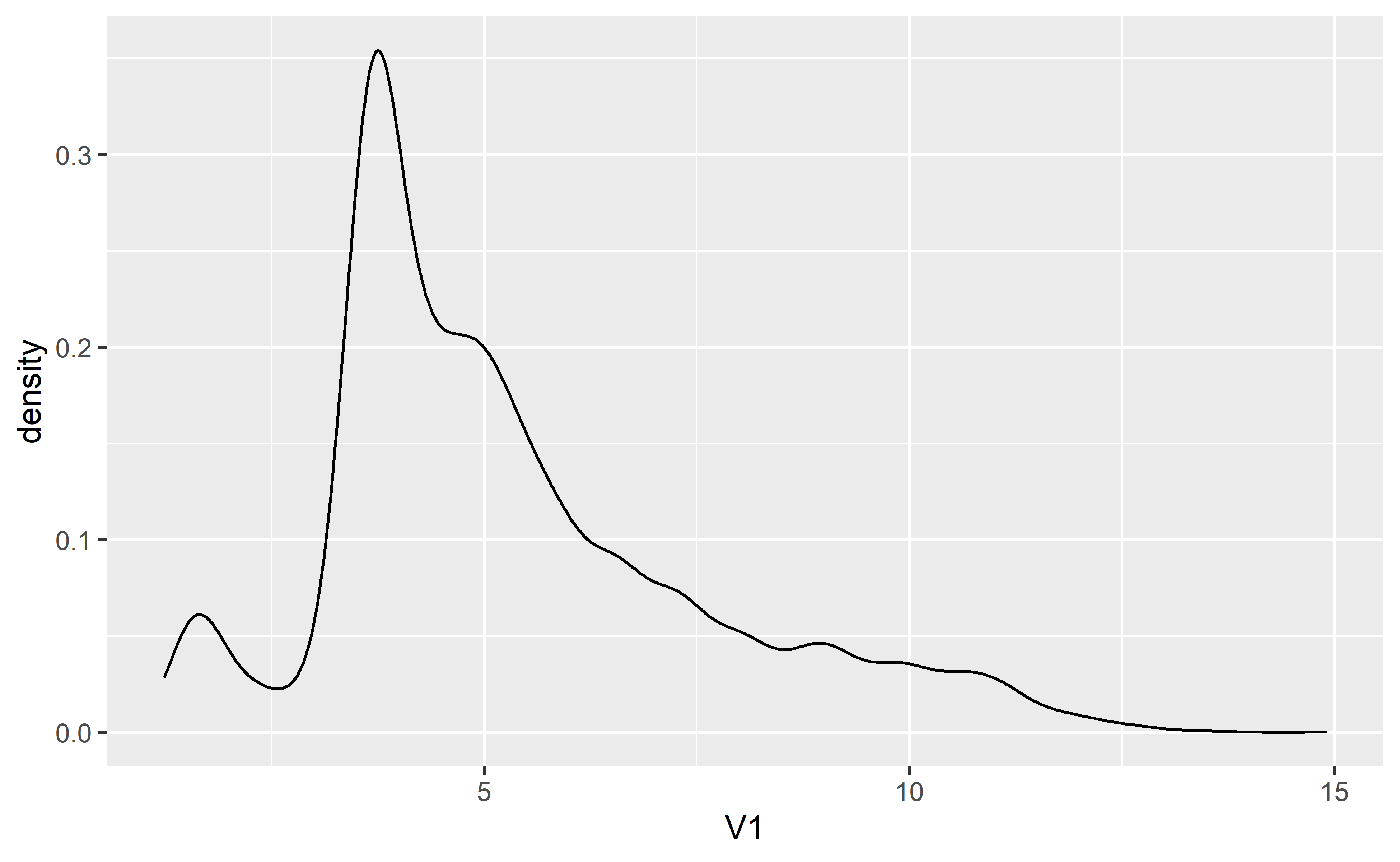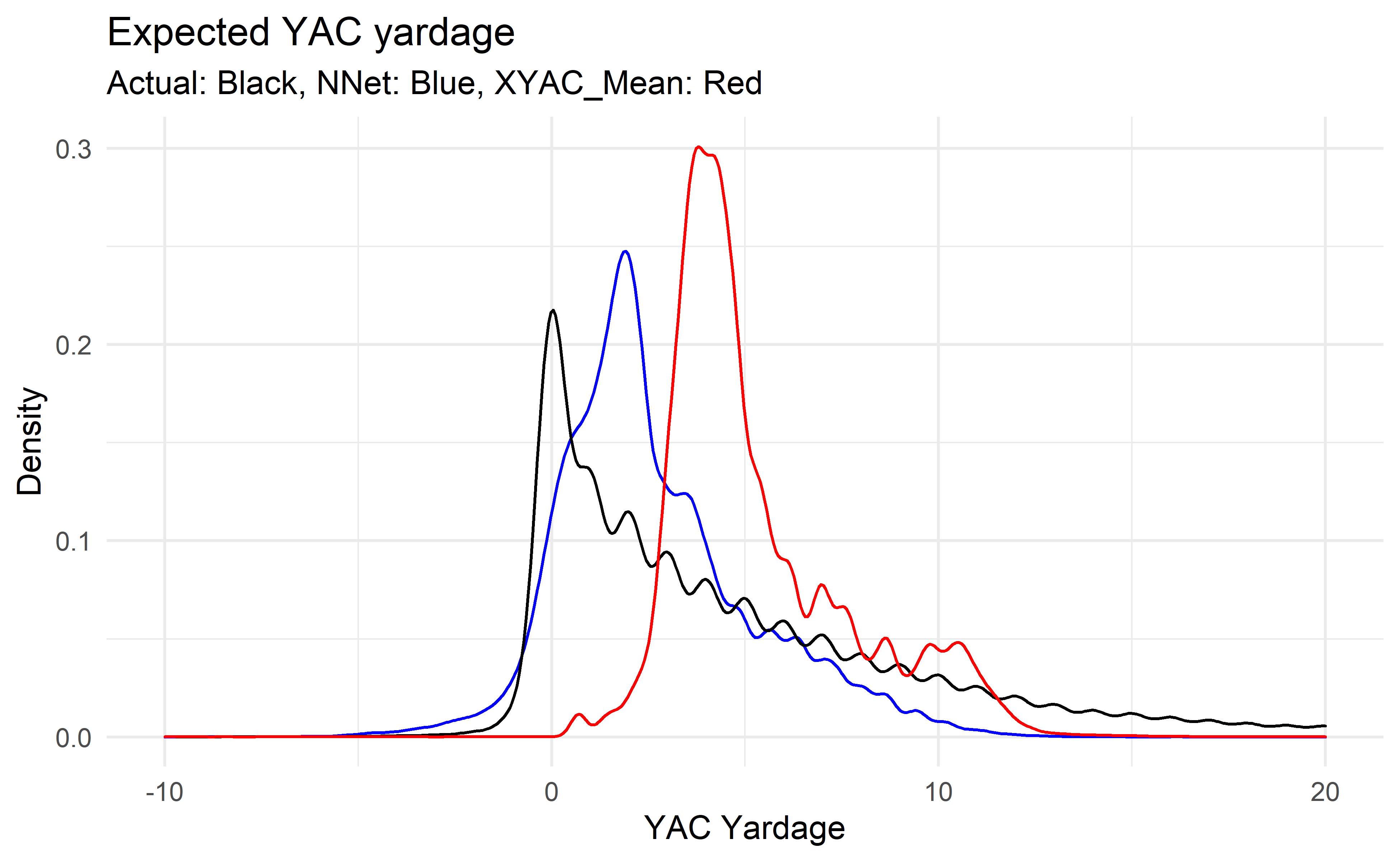# Neural Nets using R

Using Keras in R to build neural networks.

08-19-2020
``````
library(reticulate)
library(tidyverse)

use_condaenv("r-tf-gpu", required = TRUE)

library(keras)``````

Before you proceed you will need to install Keras for R. In order to do that, I followed this guide. https://github.com/antoniosehk/keras-tensorflow-windows-installation

The following guide is heavily borrowed from the following Rstudio guide! https://tensorflow.rstudio.com/tutorials/beginners/basic-ml/tutorial_basic_regression/

Use the following lines to download the data if you need to.

``````
seasons <- 2010:2019
pbp <- purrr::map_df(seasons, function(x) {
glue::glue("https://raw.githubusercontent.com/guga31bb/nflfastR-data/master/data/play_by_play_{x}.csv.gz")
)
})``````

Instead of throwing the kitchen sink at a problem, let’s choose some variables we think would influence yards after catch.

``````
df <-
pbp %>%
filter(pass == 1) %>%
mutate(
pass_location = as.numeric(ifelse(pass_location == "middle", 1, 0)),
roof = as.numeric(as.factor(roof))
) %>%
select(yardline_100, down, ydstogo, shotgun, air_yards, yards_after_catch, qb_hit, pass_location, roof) %>%
na.omit()``````

In this step you are converting your data frame into something Keras can injest.

``````
set.seed(7)
sample <- sample.int(n = nrow(df), size = floor(.9*nrow(df)), replace = F)
train_df <- df[sample, ]
test_df  <- df[-sample, ]

train_labels <- train_df\$yards_after_catch
test_labels <- test_df\$yards_after_catch

train_df <- train_df %>% select(-yards_after_catch)
test_df <- test_df %>% select(-yards_after_catch)

column_names <- colnames(train_df)

train_df <- train_df %>%
as_tibble(.name_repair = "minimal") %>%
setNames(column_names) %>%
mutate(label = train_labels)

test_df <- test_df %>%
as_tibble(.name_repair = "minimal") %>%
setNames(column_names) %>%
mutate(label = test_labels)``````

Next we’ll use a little helper function to create the model, here we’re just doing a little toy model. No convolutions or anything too fancy. Just a little good ole fashioned brute force! Mostly because you should go read about different network types before you use them. :)

``````
library(tfdatasets)

spec <- feature_spec(train_df, label ~ . ) %>%
step_numeric_column(all_numeric(), normalizer_fn = scaler_standard()) %>%
fit()

spec``````
``````
-- Feature Spec ---------------------------------------------------------------------------------------------------------------------------------------------------------------------------------------
A feature_spec with 8 steps.
Fitted: TRUE
-- Steps ----------------------------------------------------------------------------------------------------------------------------------------------------------------------------------------------
The feature_spec has 1 dense features.
StepNumericColumn: yardline_100, down, ydstogo, shotgun, air_yards, qb_hit, pass_location, roof
-- Dense features ------------------------------------------------------------------------------------------------------------------------------------------------------------------------------------- ``````
``````
layer <- layer_dense_features(
feature_columns = dense_features(spec),
dtype = tf\$float32
)

build_model <- function() {
input <- layer_input_from_dataset(train_df %>% select(-label))

output <- input %>%
layer_dense_features(dense_features(spec)) %>%
layer_dense(units = 64, activation = "relu") %>%
layer_dropout(.25) %>%
layer_dense(units = 64, activation = "relu") %>%
layer_dropout(.25) %>%
layer_dense(units = 64, activation = "relu") %>%
layer_dropout(.25) %>%
layer_dense(units = 1)

model <- keras_model(input, output)

model %>%
compile(
loss = "mse",
metrics = list("mean_absolute_error")
)

model
}

early_stop <- callback_early_stopping(monitor = "val_loss", patience = 20)

print_dot_callback <- callback_lambda(
on_epoch_end = function(epoch, logs) {
if (epoch %% 20 == 0) cat("\n")
cat(".")
}
)

model <- build_model()

summary(model)``````
``````
Model: "model"
______________________________________________________________________
Layer (type)           Output Shape   Param # Connected to
======================================================================
air_yards (InputLayer) [(None,)]      0
______________________________________________________________________
down (InputLayer)      [(None,)]      0
______________________________________________________________________
pass_location (InputLa [(None,)]      0
______________________________________________________________________
qb_hit (InputLayer)    [(None,)]      0
______________________________________________________________________
roof (InputLayer)      [(None,)]      0
______________________________________________________________________
shotgun (InputLayer)   [(None,)]      0
______________________________________________________________________
yardline_100 (InputLay [(None,)]      0
______________________________________________________________________
ydstogo (InputLayer)   [(None,)]      0
______________________________________________________________________
dense_features_1 (Dens (None, 8)      0       air_yards
down
pass_location
qb_hit
roof
shotgun
yardline_100
ydstogo
______________________________________________________________________
dense (Dense)          (None, 64)     576     dense_features_1
______________________________________________________________________
dropout (Dropout)      (None, 64)     0       dense
______________________________________________________________________
dense_1 (Dense)        (None, 64)     4160    dropout
______________________________________________________________________
dropout_1 (Dropout)    (None, 64)     0       dense_1
______________________________________________________________________
dense_2 (Dense)        (None, 64)     4160    dropout_1
______________________________________________________________________
dropout_2 (Dropout)    (None, 64)     0       dense_2
______________________________________________________________________
dense_3 (Dense)        (None, 1)      65      dropout_2
======================================================================
Total params: 8,961
Trainable params: 8,961
Non-trainable params: 0
______________________________________________________________________``````

Next let’s run the model and see how it does!

``````
history <- model %>% fit(
x = train_df %>% select(-label),
y = train_df\$label,
epochs = 500,
batchsize = 64,
validation_split = 0.2,
verbose = 0,
callbacks = list(print_dot_callback, early_stop)
)``````
``````
....................
.................``````

Now to check the results!

Here we visualize how our nnet trained over our epochs. We define epochs here since we had some early stopping.

``````
library(ggplot2)
history\$params\$epochs <- length(history\$metrics\$loss)

plot(history)````````````
test_predictions <- model %>% predict(test_df %>% select(-label))``````

Next we can take a look at the mean absolute error and loss from our model on the test set.

``````
c(loss, mae) %<-% (model %>% evaluate(test_df %>% select(-label), test_df\$label, verbose = 0))

loss``````
``````
 46.37026``````
``````
mae``````
``````
 4.239087``````
``````
test_predictions <- test_predictions %>% as.data.frame()

test_predictions %>% ggplot(aes(V1)) + geom_density()``````Lastly, let’s visualize our trained model versus both actual YAC yardage and nflfastR’s XYAC mean yards model.

``````
df <-
pbp %>%
filter(pass == 1) %>%
mutate(
pass_location = as.numeric(ifelse(pass_location == "middle", 1, 0)),
roof = as.numeric(as.factor(roof))
) %>%
select(yardline_100, down, ydstogo, shotgun, air_yards, yards_after_catch, qb_hit, pass_location, roof, xyac_mean_yardage) %>%
na.omit()

train_df <- df %>%
as_tibble(.name_repair = "minimal") %>%
setNames(colnames(df)) %>%
mutate(label = yards_after_catch)

test_predictions <- model %>% predict(train_df %>% select(-label))

test_predictions <- test_predictions %>% as.data.frame()

df <-
cbind(df, test_predictions)

df %>%
ggplot() +
geom_density(aes(V1), color = "blue") +
geom_density(aes(yards_after_catch)) +
geom_density(aes(xyac_mean_yardage), color = "red") +
xlim(c(-10, 20)) +
theme_minimal() +
labs(
title = "Expected YAC yardage",
x = "YAC Yardage",
y = "Density",
subtitle = "Actual: Black, NNet: Blue, XYAC_Mean: Red"

)``````There you have it, your own little nnet done completely in R using the Keras/tensorflow backend.

### Corrections

If you see mistakes or want to suggest changes, please create an issue on the source repository.

### Reuse

Text and figures are licensed under Creative Commons Attribution CC BY-NC 4.0. Source code is available at https://github.com/nflverse/open-source-football, unless otherwise noted. The figures that have been reused from other sources don't fall under this license and can be recognized by a note in their caption: "Figure from ...".

### Citation

`Darkweb (2020, Aug. 19). Open Source Football: Neural Nets using R. Retrieved from https://www.opensourcefootball.com/posts/2020-08-19-neural-nets-using-r/`
```@misc{darkweb2020neural,